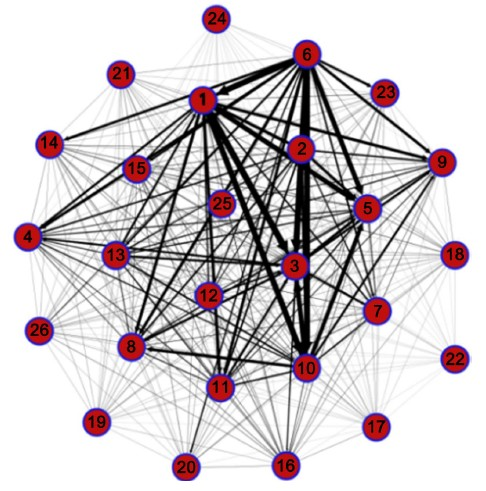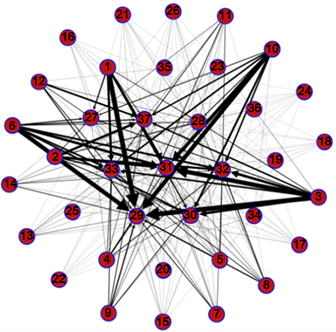﻿ 系统重要性银行与系统重要性行业分析——基于复杂网络的研究 Systematic Important Banks and Systematic Important Industries—Research on Complex Network

Finance
Vol. 09  No. 03 ( 2019 ), Article ID: 30161 , 8 pages
10.12677/FIN.2019.93023

Systematic Important Banks and Systematic Important Industries

—Research on Complex Network

Qiaomei Bian, Yonghui Zhai, Xiaobo She

Business School of Henan Normal University, Xinxiang HenanReceived: Apr. 21st, 2019; accepted: May 6th, 2019; published: May 13th, 2019ABSTRACT

Based on the maximum and minimum cross-entropy principle, this paper simulates the micro-loan data of the interbank market in 2015. Combining with the actual loan data of the corresponding bank-entity industry, the paper draws the inter-bank network and the inter-bank-entity industry network respectively with Gephi, from the perspective of economic and financial related network. According to the statistical theory of complex networks, the paper identifies and analyzes the systemic importance banks, the systemic importance industries and the internal relationship between them, taking the degree, weighted average degree and median centrality as the measurement indicators. It is hoped that this paper will provide some guidance for the supervisory authorities to focus on the key links of the whole economic system from the overall perspective, control system risks and reduce management costs.

Keywords:Complex Network, Minimum Cross Entropy, Systematic Important Bank, Systematic Importance Industry

——基于复杂网络的研究Copyright © 2019 by authors and Hans Publishers Inc.1. 引言

2008年的次贷危机和2010年的欧债危机表明，在高度关联的金融体系，个别机构的破产或衰退，可能会通过千丝万缕的业务联系，产生高风险的“多米诺骨牌”效应，引发系统性风险，最终加剧实体经济的衰退。2019年初，习近平总书记曾说过，实体经济是肌体，金融体系是血脉，要使金融业更好地为实体经济服务，做到稳增长，控风险。可见，金融行业与实体经济是休戚与共的关系。

2. 模型与数据

2.1. 银行间市场债权债务结构的估计模型

$\begin{array}{l}{X}_{N×N}\left[\begin{array}{lllll}{x}_{ij}\hfill & \cdots \hfill & {x}_{1j}\hfill & \cdots \hfill & {x}_{1N}\hfill \\ ⋮\hfill & \ddots \hfill & ⋮\hfill & ⋰\hfill & ⋮\hfill \\ {x}_{i1}\hfill & \cdots \hfill & {x}_{ij}\hfill & \cdots \hfill & {x}_{iN}\hfill \\ ⋮\hfill & ⋰\hfill & ⋮\hfill & \ddots \hfill & ⋮\hfill \\ {x}_{N1}\hfill & \cdots \hfill & {x}_{Nj}\hfill & \cdots \hfill & {x}_{NN}\hfill \end{array}\right]\begin{array}{l}{a}_{1}\hfill \\ ⋮\hfill \\ {a}_{i}\hfill \\ ⋮\hfill \\ {a}_{N}\hfill \end{array}\\ \text{}{\sum }_{i}{l}_{1}\cdots {l}_{j}\cdots {l}_{N}\end{array}$ (1)

$\begin{array}{l}{X}_{N×N}^{*}\left[\begin{array}{lllll}0\hfill & \cdots \hfill & {x}_{1j}^{*}\hfill & \cdots \hfill & {x}_{1N}^{*}\hfill \\ ⋮\hfill & \ddots \hfill & ⋮\hfill & ⋰\hfill & ⋮\hfill \\ {x}_{i1}^{\text{*}}\hfill & \cdots \hfill & 0\hfill & \cdots \hfill & {x}_{ij}^{*}\hfill \\ ⋮\hfill & ⋰\hfill & ⋮\hfill & \ddots \hfill & ⋮\hfill \\ {x}_{N1}^{\text{*}}\hfill & \cdots \hfill & {x}_{{}_{Nj}}^{\text{*}}\hfill & \cdots \hfill & 0\hfill \end{array}\right]\begin{array}{l}{a}_{1}\hfill \\ ⋮\hfill \\ {a}_{i}\hfill \\ ⋮\hfill \\ {a}_{N}\hfill \end{array}\\ \text{}{\sum }_{i}{l}_{1}\cdots {l}_{j}\cdots {l}_{N}\end{array}$ (2)

$\mathrm{min}\underset{i=1}{\overset{N}{\sum }}\underset{j=1}{\overset{N}{\sum }}{X}_{ij}^{*}\mathrm{ln}\frac{{x}_{ij}^{*}}{{x}_{ij}^{*}}$ (3)

$\underset{j=1}{\overset{N}{\sum }}{x}_{ij}={a}_{i},\underset{i=1}{\overset{N}{\sum }}{x}_{ij}={l}_{i},0*\mathrm{ln}\frac{0}{0}=0,{x}_{ij}^{*}\ge 0$

2.2. 样本数据选取Figure 1. Interbank network diagramFigure 2. Bank-entity network diagram

3. 复杂网络分析

3.1. 银行间网络分析

Table 2. Ranking of systematic important banks

3.2. 银行–实体行业间的网络分析Table 3. Ranking of systematic importance industries

3.3. 系统重要性银行与系统重要性行业的内部关联分析Table 4. Ranking of loans from banks to entity industries

4. 结论

Systematic Important Banks and Systematic Important Industries—Research on Complex Network[J]. 金融, 2019, 09(03): 197-204. https://doi.org/10.12677/FIN.2019.93023

1. 1. Allen, F. and Babus, A. (2009) Networks in Finance. In: Kleindorfer, P.R. and Wind, Y., Eds., The Network Challenge: Strategy, Profit, and Risk in an Interlinked World, Prentice Hall Professional, Upper Saddle River, NJ, 367-382.

2. 2. Bech, M., Chapman, J. and Garratt, R. (2008) Which Bank Is the “Central”Bank? An Application of Markov Theory to the Canadian Large Value Transfer System. Federal Reserve Bank of New York Staff Report No. 356. https://doi.org/10.2139/ssrn.1310283

3. 3. 高国华, 潘英丽. 基于资产负债表关联的银行系统性风险研究[J]. 管理工程学报, 2012, 26(4): 162-168.

4. 4. 欧阳红兵, 刘晓东. 中国金融机构的系统重要性及系统性风险传染机制分析——基于复杂网络的视角[J]. 中国管理科学, 2015, 23(10): 30-37.

5. 5. 邓向荣, 曹红. 系统性风险、网络传染与金融机构系统重要性评估[J]. 中央财经大学学报, 2016(3): 52-60.

6. 6. 陈少炜, 李旸. 我国银行体系的网络结构特征——基于复杂网络的实证分析[J]. 经济问题, 2016(8): 56-63.

7. 7. 方意, 张子文. 系统性风险在银行间的传染路径研究——基于持有共同资产网络模型[J]. 国际金融研究, 2016, 350(6): 61-72.

8. 8. Blien, U. and Grae, F (1997) Entropy Optimizing Methods for the Estimation of Tables. In: Balderjahn, I., Mathar, R. and Schader, M., Eds., Classification, Data Analysis and Data Highways, Springer Verlag, Berlin.